# Infosys Puzzle Solving Previous Year Questions

28 January 2023

Infosys Puzzle Solving Previous Year Questions

Infosys Puzzle Solving Questions

Q1) Given is the set of nine numbers that relate to each other in a certain way.

Determine the missing number.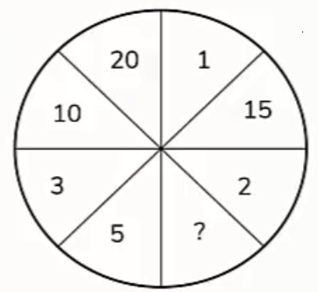A. 4

B. 7

C. 3

D. 90

Explanation: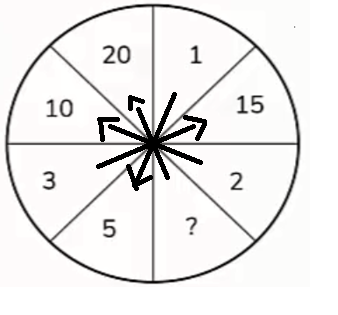1* 5 = 5

2*5 = 10

3*5 = 15

Hence 4* 5 = 20

Therefore, ? = 4

Q2) Find the missing terms in the third figure. Assume the third figure works in the same way as the other two.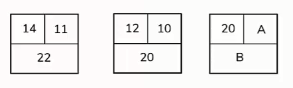A. A=9,B=17

B. A=14,B=22

C. A=14,B=28

D. A=18,B=9

Explanation: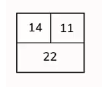14 +8 = 22

22/2 = 11

Similarly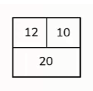12+8 = 20

20/2 = 10

Hence,20 + 8 = 28 (B)

28/2 = 14 (A)

A = 14 and B = 28

Q3) Find the missing term in the third figure. Assume the third figure works in the same way as the other two.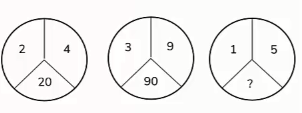A. 50

B. 110

C. 61

D. 26

Explanation:

Figure 1 -- 2, 4 & 20

which is 2×2+4×4=20

Figure 2 -- 3,9 & 90

which is 3×3+9×9=90

therefore, 1,5=1×1+5×5=26

Q4) Which number should replace the question mark in the series: 14, 17, 22, 29, ?

A. 40

B. 39

C. 41

D. 43

Explanation:

14 +3 = 17

17 + 5 =22

22+7 = 29

Therefore, the next number will be 29+11 = 40

Q5) If sum of digits of a number 3Q6 is 9 then Q is ____.

A. Three

B. Two

C. One

D. Zero

Explanation:

If sum of digits of a number 3Q6 is 9 then Q is 0

3 + Q + 6 = 9

Q = 0

Q6) If X + X + X =33; X + Y + Y = 31 then X + Y = ?

A. 21

B. 18

C. 16

D. 12

Explanation:

If X + X + X =33

3X=33

X = 11

Sub X=11

X + Y + Y = 31

2Y +11 = 31

2Y = 20

Y = 10

then X + Y = 11+10 = 21

Q7) The missing number in the sequence : 25, 36, 49, ?, 81 is ___.

A. 64

B. 60

C. 72

D. 68

Explanation:

The missing number in the sequence 25, 36, 49,?, 81 is 64

25 = 52

36 = 62

49 = 72

64 = 82

81 = 92

####